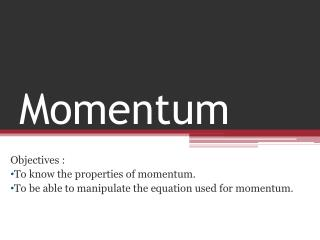DownloadDownload PresentationMomentum

# Momentum

Download Presentation## Momentum

- - - - - - - - - - - - - - - - - - - - - - - - - - - E N D - - - - - - - - - - - - - - - - - - - - - - - - - - -
##### Presentation Transcript

1. Momentum Objectives : To know the properties of momentum. To be able to manipulate the equation used for momentum.

2. Starter: • Write down the unit of each of the following in your notebook: • a= • d= • v= • t= • m= • F=

3. Momentum Momentum = mass x velocity p=mv Units? Unit of p = unit of m X unit of V = kg X m/s = kgm/s or = kg m s‾¹

4. Momentum • Is a vector quantity so direction is important (ie can be negative) • In any collision or interaction Momentum is always conserved

5. Momentum is always conserved: The total momentum of two objects before they collide is equal to the total momentum after the collision. momentum before = momentum after p1 + p2 = ptotal 300kgms-1 100kgms-1 200kgms-1

6. Example 1: A skater of mass 75kg and velocity of 4ms-1 collides with a stationary skater of mass 50kg. Calculate their velocity after impact. 75kg X 4ms-1 =300kgms-1 Momentum before collision = + 0 Momentum after collision = (75 + 50) kg X v 300kgms-1 = (75 + 50) kg X v v = 300kgms-1 /(75 + 50) kg v = 2.4ms-1

7. Example 2: A bullet of mass 5 g is shot from a rifle at a speed of 200ms-1 The rifle has a mass of 4kg. Calculate the velocity at which the rifle recoils. Momentum before firing = 0 Momentum after firing= Momentum of bullet + momentum of rifle = 0 + 4 kg×(v)=0  0.005 kg × 200ms-1  1kgms-1+4kgv=0  v= - 1kg ms-1 /4kg v = -0.25ms-1 Since, v= -0.25 ms-1 then the velocity of the rifle was in the opposite direction of the velocity of the bullet.

8. Why is it true? • In any collision the force between them is the same and acts for the same time (Newton’s 3) • This force acts on different objects however and will accelerate each object differently according to the mass of each (Newton’s 2 ) • The final velocity reached by each object will decrease in the same proportion as the mass of the objects increases • The product m x v for each object is therefore identical

9. Why is this useful? • It allows us to predict the outcome of collisions eg car rail and air accident simulations and construct vehicles to stand up to them better • It allows us to calculate the speed of objects which would otherwise be difficult eg the speed of a shell or rifle bullet • It might allow us to become excellent snooker players but……..

10. Try these….. • 1. A trolley of mass 4kg moving at 10 m/s collides with a 2 kg trolley moving in the same direction at a velocity of 4m/s. they separate after the collision and the 4 kg trolley slows to 7m/s. What is the final speed of the other trolley? (hint draw diagrams of momentum before and after) • 2. a trolley A of mass 1kg is travelling at 2m/s towards another trolley B of mass 4kg which is travelling towards it at a velocity of 3m/s. On collision they stick together. What is their final combined velocity and in which direction do they travel together? (care with negatives) 10m/s 2m/s to the left

11. 1. What is the momentum in kg.m.s-1 of a 10 kg truck traveling at • i. 5 m.s-1 ii. 20 cm.s-1 iii. 36 km.h-1 • 2. Jack, whose mass is 48 kg, is running at 5 m.s-1 and jumps onto a 7 kg skateboard moving in the same direction at 3.0 m.s-1. What is their speed after Jack is on the skateboard? • 3. A truck of mass 500 kg moving at 4 m.s-1east collides with another truck of mass 1500 kg moving at 1.5 m.s-1west. What is their common velocity after the collision if they stick together? (Hint: Make one of the velocities negative) • Difficult question for physics geniuses only • 4. A comet of mass 1 x 106 kg hits the earth at a speed of 1 x 105 m.s-1. At what speed does the earth move backwards after the collision (mass of earth = 700 x 1022 kg)? (Assume that the earth is not moving before the collision, a big assumption!)